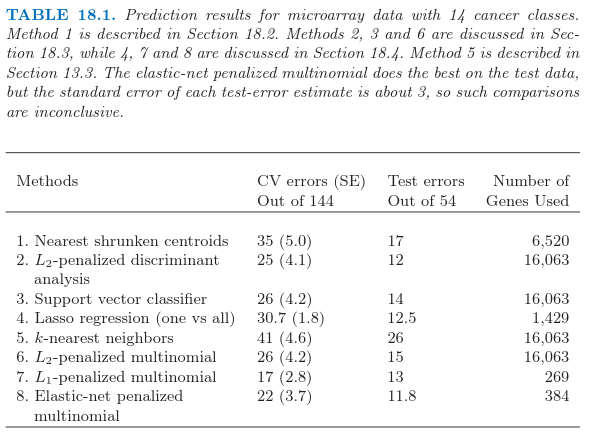# 18.3 二次正则化的线性分类器¶

Ramaswamy et al. (2001)1提出一个更加困难的微阵列分类问题，涉及 144 个病人的 14 种癌症类型的训练集，以及含有 54 个病人的测试集．已知 16063 个基因的表达值．RDA（正则判别分析），正则多元逻辑斯蒂回归，以及支持向量机是更复杂的方法，试图研究数据的多变量信息．我们依次描述每个方法，以及一系列正则化方法，包括 $L_1$ 和 $L_2$，以及两个都有的方法．

Recall

Recall

weiya 注：Recall

weiya 注：Ex. 18.3

## 支持向量分类器¶

weiya 注：Recall

Tibshirani and Hastie (2007)8提出 边际树 (margin tree) 分类器，这是支持向量分类器用在二叉树中，很像在 CART（第 9 章）中一样．类别以分层形式进行组织，举个例子，对于将病人分类成不同的癌症类型是很有用的．

weiya 注：Recall

## 当 $p > > N$ 时的计算捷径¶

Ex. 18.4

Ex. 18.6

Ex. 18.12

12.3.7 节中的支持向量机的“核技巧”在不同的情形下利用了这节中的降维．假设我们有 $N\times N$ 的（内积）矩阵 $\mathbf{K=XX^T}$．由 \eqref{18.12} 我们有 $\mathbf{K=UD^2U^T}$，所以 $\mathbf K$ 捕捉了与 $\mathbf R$ 一样的信息．练习 18.13 显示了我们怎样利用这节的想法从 $\mathbf K$ 和它的 SVD 分解来拟合岭逻辑斯蒂回归．

Ex. 18.13

1. Ramaswamy, S., Tamayo, P., Rifkin, R., Mukherjee, S., Yeang, C., Angelo, M., Ladd, C., Reich, M., Latulippe, E., Mesirov, J., Poggio, T., Gerald, W., Loda, M., Lander, E. and Golub, T. (2001). Multiclass cancer diagnosis using tumor gene expression signature, PNAS 98: 15149–15154.

2. Guo, Y., Hastie, T. and Tibshirani, R. (2006). Regularized linear discriminant analysis and its application in microarrays, Biostatistics 8: 86–100.

3. Zhu, J. and Hastie, T. (2004). Classification of gene microarrays by penalized logistic regression, Biostatistics 5(2): 427–443.

4. Friedman, J., Hastie, T. and Tibshirani, R. (2010). Regularization paths for generalized linear models via coordinate descent, Journal of Statistical Software 33(1): 1–22.

5. Rosset, S., Zhu, J. and Hastie, T. (2004a). Boosting as a regularized path to a maximum margin classifier, Journal of Machine Learning Research 5: 941–973.

6. Vapnik, V. (1998). Statistical Learning Theory, Wiley, New York.

7. Weston, J. and Watkins, C. (1999). Multiclass support vector machines, in M. Verleysen (ed.), Proceedings of ESANN99, D. Facto Press, Brussels.

8. Tibshirani, R. and Hastie, T. (2007). Margin trees for high-dimensional classification, Journal of Machine Learning Research 8: 637–652.

9. Hastie, T. and Tibshirani, R. (2004). Efficient quadratic regularization for expression arrays, Biostatistics 5(3): 329–340. 下载